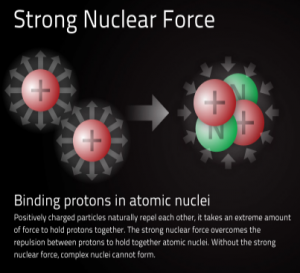Home » Physics » Nuclear Force – Definition | properties of Nuclear Force

# Nuclear Force – Definition | properties of Nuclear Force

Question We already know about gravitational and electrostatic forces now a question arises that does these forces still hold when we talk about nuclear dimensions as gravitational force between two protons is far too less to hold them and electrostatic force between two protons always a force repulsive in nature . So what hold protons together to form a nucleus.

Answer is Nuclear force which are attractive in nature and strong enough to overcome the electrostatic force of repulsion between two protons. So it is the nuclear forces which holds neutrons and protons (nucleons) together to form a nucleus. Nucleus forces are complex in nature and difficult to understand.Some of the properties of nuclear forces are given below.
(1) Nuclear forces are strong attractive forces :- These are strongest forces and are about 100 times stronger then electrostatic forces and about 1038 times stronger then gravitational forces. These are the forces which holds nucleons in a nucleus.
(2) Nuclear forces are short range forces :- The nuclear forces are most effective up to a distance of the order of 10-15m or less. These forces decreases rapidly as the separation distance between nucleons increases and becomes negligible when separation between nucleons is of the order of 10 fermi . The effective range up to which nuclear forces are effective is known as nuclear range.
(3) Nuclear forces are the charge independent :- Nuclear forces acting between two protons , two neutrons and between a proton and neutron have same strength and the nature of force is attractive.
(4) Nuclear forces are not central forces :- These forces do not obey inverse square law. The magnitude of nuclear force depends on the direction of spin of nucleons.
(5) Nuclear forces are saturated forces :- A nucleon experiences the force only due to it nearest neighboring nucleons.

Subscribe
Notify of

This site uses Akismet to reduce spam. Learn how your comment data is processed.